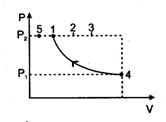Consider the following reactions :

(i)

(ii)

(iii)

(iv)

Enthalpy of formation of H2O(l) is:

(a) -x2 kJ mol-1

(b) +x3 kJ mol-1

(c) -x4 kJ mol-1

(d) -x1 kJ mol-1

Concept Questions :-

Enthalpy and It's Type
High Yielding Test Series + Question Bank - NEET 2020

Difficulty Level:

Given those bond energies of H-H and Cl-Cl are 430 kJ mol-1 and 240 kJ mol-1 respectively and ΔHf for HCI is -90 kJ mol-1. Bond enthalpy of HCl is:

1. 290 kJ mol-1

2. 380 kJ mol-1

3. 425 kJ mol-1

4. 245 kJ mol-1

Concept Questions :-

Enthalpy and It's Type
High Yielding Test Series + Question Bank - NEET 2020

Difficulty Level:

Identify the correct statement for change of Gibbs energy for a system ($∆$Gsystem) at constant temperature and pressure:

(a) If $∆$Gsystem > 0, the process is spontaneous

(b) If $∆$Gsystem = 0, the system has attained equilibrium

(c) If $∆$Gsystem = 0, the system is still moving in a particular direction

(d) If $∆$Gsystem < 0, the process is not spontaneous

Concept Questions :-

Gibbs Energy Change
High Yielding Test Series + Question Bank - NEET 2020

Difficulty Level:

The enthalpy and entropy change for the reaction :

Br2(l)+Cl2(g) $\to$ 2BrCl(g) are 30 kJ mol-1 and 105 JK-1 mol-1 respectively.

The temperature at which the reaction will be in equilibrium is :

1. 285.7 K

2. 273 K

3. 450 K

4. 300 K

Concept Questions :-

Spontaneity and Entropy
High Yielding Test Series + Question Bank - NEET 2020

Difficulty Level:

Consider the reactionat 300K

H2(9) + Cl2(9) →2HCI(g), ΔH° = — 185 KJ

If 3 mole of H completely react with 3 mol of Cl2 to form Cl, $∆$U° of the reaction will be

(1) Zero

(2) –185 KJ

(3) -555 KJ

(4) None

Concept Questions :-

Internal Energy and work done
High Yielding Test Series + Question Bank - NEET 2020

Difficulty Level:

Fora perfectly crystalline solid Cpm = aT3, where a is constant. If Cpm is 0.42 J/K–mol at 10 K, molar entropy at 10 K is

(1) 0.42 J/K–mol

(2) 0.14 J/K–mol

(3) 4.2 J/K–mol

(4) zero

High Yielding Test Series + Question Bank - NEET 2020

Difficulty Level:

One mole of an ideal monoatomic gas expands isothermally against constant external pressure of 1 atm from initial volume of 1L to a state where its final pressure becomes equal to external pressure. If initial temperature of gas is 300 K then total entropy change of system in the above process is :

[R = 0.082 L atm mol–1 K–1 = 8.3 J mo1–1K–1].

(1) 0

(2) Rln (24.6)

(3) Rln (2490)

(4) $\frac{3}{2}$Rln(24.6)

Concept Questions :-

Spontaneity and Entropy
High Yielding Test Series + Question Bank - NEET 2020

Difficulty Level:

At 1000 K water vapour at 1 atm. has been found to be dissociated into H2 and O2 to the extent of 3 x 10–6 %.Calculate the free energy decrease of the system, assuming ideal behaviour.

(1) –ΔG = 90,060 cal

(2) –ΔG = 20 cal

(3) –ΔG = 480 cal

(4) –ΔG = –45760 cal

Concept Questions :-

Spontaneity and Entropy
High Yielding Test Series + Question Bank - NEET 2020

Difficulty Level:

When 1 mole of an ideal gas at 20 atm pressure and 15 L volume expands such that the final pressure becomes 10 atm and the final volume become 60 L. Calculate entropy change for the process (Cpm = 30.96 J mole–1 K–1)

(1) 80.2 J.klmo1–1

(2) 62.42 kJ.k–1 mol–1

(3) 120 x 102 J1c1mol–1

(4) 27.22 J.k–1mor

Concept Questions :-

CP and CV and Relation for Real Gas
High Yielding Test Series + Question Bank - NEET 2020

Difficulty Level:

An ideal gas is taken from the same initial pressure P1 to the same final pressure P2 by three different processes. If it is known that point 1 corresponds to a reversible adiabatic and point 2 corresponds to a single stage adiabatic then(1) Point 3 may be a two stage adiabatic.

(2) the average K.E. of the gas is maximum at point 1

(3) Work done by surrounding in reaching point number '3' will be maximum

(4) If point4 and point 5 lie along a reversible isotherm then T5 < T1.

Concept Questions :-

Internal Energy and work done